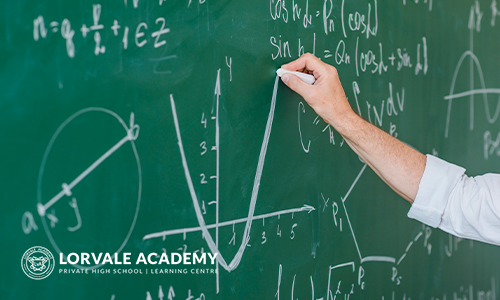# SINGAPORE MATH

Singapore math uses problem-solving as a central approach to mathematics. In this course, students of levels 1-3 will be introduced to addition, subtraction, multiplication, division, measurements, time, and money. They will learn basic number sense, patterns, and practice addition, subtraction, multiplication and division skills. Students in levels 4-7 will explore deeper into graphs and data representation, fractions, geometry, angles and lines, composite figures, 2D and 3D figures, and algebra. Applications of these concepts will be evaluated through homework on a regular basis, midterm and final tests.